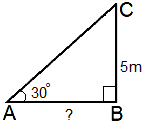# The length of the shadow is of 5 m height tree whose angle of elevation of the sun is 30° is A) 5 m  B) √3 m

31 views

The length of the shadow is of 5 m height tree whose angle of elevation of the sun is 30° is

A) 5 m

B) √3 m

C) 5 √3 m

D) 10 m

+1 vote
by (34.0k points)

Correct option is: C) 5 √3 m

by (12.6k points)
edited ago

Correct option is: C) 5 √3 mGiven that angle of elevation of the sun is $30^\circ$.

i.e, $\angle$CAB = $\angle$A = $30^\circ$

Height of the tree is BC = 5 cm.

In right $\triangle$ ABC,

tan A = $\frac {BC}{AB}$

$\frac {BC}{AB}$ = tan $30^\circ$ = $\frac {1}{\sqrt3}$ ($\because$ $\angle A=30^\circ$)

$\Rightarrow AB=BC\sqrt 3=5\sqrt3m(\because BC=5m)$

Hence, the length of the shadow of the tree is $5 \sqrt3$ m.